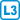## Recursive sequences

Show that the following recursively defined sequences $$\{a_n\}$$ have limits, and determine them.

• #### Variant 1

$$a_1=\sqrt c$$, where $$c$$ is a positive real number, and $$a_{n+1}=\sqrt{a_n+c}$$.

• #### Variant 2

$$a_1=0$$ and $$a_{n+1}=a_n+\frac12 (x-a_n)^2$$, for $$0\le x \le 1$$.

• #### Variant 3

$$a_1=\sqrt{2}$$ and $$a_{n+1}=\sqrt{2-a_n}$$.

• #### Variant 4

$$a_1=1$$ a $$a_{n+1}=\frac1{1+a_n}$$

• #### Variant 5

$$a_1= c$$, where $$c$$ is a positive real nuimber, and $$\displaystyle a_{n+1}=\frac12\left(a_n+\frac2{a_n}\right)$$.